# Detect and remove loop in a linked list

Problem Statement: Given the head of a linked list, determine if the linked list has a cycle in it and remove it.

Examples:

```Input: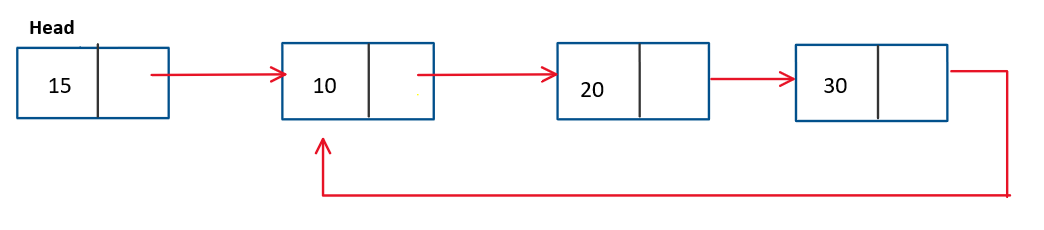Output: Cycle exists.```

The final linked list is as follows after removing the loop: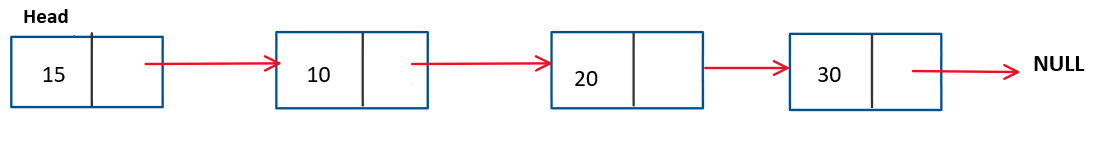### Solution

DisclaimerDon’t jump directly to the solution, try it out yourself first.

We can divide this problem into three sub-problems:

1. Detecting a cycle in linked list
2. Starting point of the cycle in linked list
3. Removing the cycle in linked list

Let’s solve each sub-problem one by one and combine to get the final solution

## Solution 1:

Approach: BruteForce Approach

We can use hashing. Following steps are involved in this approach:

1. Traverse every node in the linked list and insert them in the hashtable.
2. Maintain a prev pointer which keeps the track of previous nodes..
3. If a node is already present in the hashtable, this indicates that the loop is present.
4. To break the loop, make the next pointer of the prev pointer to point to NULL.

Let’s understand this approach from the code given below

Code:

## C++ Code

``````#include<iostream>
#include<unordered_set>
using namespace std;

struct node {
int data;
node * next;
node(int d) {
data = d;
next = NULL;
}
};
void loopDetDelBrute(node * head) {
unordered_set < node * > uns;

node * prev = NULL;
node * current = head;
while (current != NULL) {
if (uns.find(current) != uns.end()) { // loop detected
prev -> next = NULL; // breaking the loop
return;
}
uns.insert(current);

prev = current;
current = current -> next;
}
}
// for checking if our approach works
bool FLD(node * head) {
node * slow = head;
node * fast = head;

while (fast != NULL && fast -> next != NULL) {
slow = slow -> next;
fast = fast -> next -> next;
if (slow == fast) return true;
}
return false;
}
int main() {
node * head = new node(15);
head -> next = new node(10);
head -> next -> next = new node(12);
head -> next -> next -> next = new node(20);
head -> next -> next -> next -> next = head -> next;
FLD(head) ? cout << "Loop Detected" : cout << "Loop not detected";
}
``````

Time complexity: O(n)

Space complexity: O(n)

## Java Code

``````import java.util.HashSet;
import java.util.Set;

class node {
int data;
node next;
node(int d) {
data = d;
next = null;
}
}

class Main {

public static void main(String args[]) {
node head = new node(15);
head.next = new node(10);
head.next.next = new node(12);
head.next.next.next = new node(20);
System.out.println("Loop Detected");
} else System.out.println("Loop not detected");
}

static void loopDetDelBrute(node head) {
Set < node > set = new HashSet < > ();
node prev = null;
node current = head;
while (current != null) {
if (set.contains(current)) // loop detected
{
prev.next = null; // loop removal
return;
}

prev = current;
current = current.next;
}

}
// for checking if our approach is correct
public static boolean FLD(node head) {
node slow = head;
node fast = head;
while (fast != null && fast.next != null) {
slow = slow.next;
fast = fast.next.next;
if (slow == fast) return true;
}
return false;
}
}
``````

Time complexity: O(n)

Space complexity: O(n)

### Solution 2:

Approach: Better Approach

In this approach, we count the number of nodes in the loop and then perform the necessary operations to remove the loop.

1. Use Floyd’s cycle detection algorithm to check for the cycle.
2. Now, we count the number of nodes (x) which are a part of the loop.
3. Now, we use two pointers, one placed at the head node and other placed at xth node from the head.
4. Now we move both the pointers at the same speed and wherever they meet, that would be the starting node of our loop.
5. Now we point the last node of the loop to NULL.

Let’s check the code for this approach for a better understanding

Code:

## C++ Code

``````#include<iostream>
using namespace std;

struct node {
int data;
node * next;
node(int d) {
data = d;
next = NULL;
}
};
void loopDetDelBetter(node * head) {
// step 1
node * slow = head, * fast = head;
while (fast != NULL && fast -> next != NULL) {
slow = slow -> next;
fast = fast -> next -> next;
if (slow == fast) break; // first meeting point
}
if (slow != fast) return;

node * ptr1 = slow;
node * ptr2 = slow;

// step 2
int x = 1;
while (ptr2 -> next != ptr1) {
ptr2 = ptr2 -> next;
x++;
}

// step 3
ptr1 = head; // move one pointer to head

// move one pointer to xth node from head
for (int i = 0; i < x; i++) {
ptr2 = ptr2 -> next;
}

// step 4
while (ptr1 != ptr2) {
ptr1 = ptr1 -> next;
ptr2 = ptr2 -> next;
}

// step 5
while (ptr2 -> next != ptr1) {
ptr2 = ptr2 -> next;
}
ptr2 -> next = NULL;
}
// to check if our approach is correct
bool FLD(node * head) {
node * slow = head;
node * fast = head;

while (fast != NULL && fast -> next != NULL) {
slow = slow -> next;
fast = fast -> next -> next;
if (slow == fast) return true;
}
return false;

}
int main() {
node * head = new node(15);
head -> next = new node(10);
head -> next -> next = new node(12);
head -> next -> next -> next = new node(20);
head -> next -> next -> next -> next = head -> next;
FLD(head) ? cout << "Loop Detected" : cout << "Loop not detected";
}
``````

Time Complexity: O(n)

Space Complexity: O(1)

## Java Code

``````class node {
int data;
node next;
node(int d) {
data = d;
next = null;
}
}

class Main {

public static void main(String args[]) {
node head = new node(15);
head.next = new node(10);
head.next.next = new node(12);
head.next.next.next = new node(20);
System.out.println("Loop Detected");
} else System.out.println("Loop not detected");
}

static void loopDetDelBetter(node head) {
// step 1
node slow = head;
node fast = head;

while (fast != null && fast.next != null) {
slow = slow.next;
fast = fast.next.next;
if (slow == fast) {
break;
}
}
if (slow != fast) return;

node ptr1 = slow;
node ptr2 = slow;

// step 2
int x = 1;
while (ptr2.next != ptr1) {
ptr2 = ptr2.next;
x++;
}

// step 3
ptr1 = head; // move one pointer to head
for (int i = 0; i < x; i++) {
ptr2 = ptr2.next; // move one pointer to xth node from head;
}

// step 4
while (ptr1 != ptr2) {
ptr1 = ptr1.next;
ptr2 = ptr2.next;
}

// step 5
while (ptr2.next != ptr1) {
ptr2 = ptr2.next;
}
ptr2.next = null;
}
public static boolean FLD(node head) {
node slow = head;
node fast = head;
while (fast != null && fast.next != null) {
slow = slow.next;
fast = fast.next.next;
if (slow == fast) return true;
}
return false;
}
}
``````

Time Complexity: O(n)

Space Complexity: O(1)

### Solution 3:

Approach: Optimal Approach

In this approach, we don’t count the number of nodes in the loop

Following are the steps to be performed while implementing this approach

1. Use floyd detection cycle to detect the cycle in the linked list
2. Move the slow pointer to the head node.
3. Now move the fast and the slow pointer with the same speed.
4. Wherever they meet, that is the starting node of the cycle.
5. Change the next pointer of the previous node to point to NULL thus breaking the cycle present in the linked list.

Let’s check the execution of this approach

Code:

## C++ Code

``````#include<iostream>
using namespace std;

struct node {
int data;
node * next;
node(int d) {
data = d;
next = NULL;
}
};

void loop_detection_deletion(node * head) {

// Floyd Loop Detection Algorithm
node * slow = head, * fast = head;
while (fast != NULL && fast -> next != NULL) {
slow = slow -> next;
fast = fast -> next -> next;
if (slow == fast) break; // first meeting point
}
if (slow != fast) return;

// Loop Removal Algorithm
// locating the starting node of the loop
while (slow -> next != fast -> next) {
slow = slow -> next;
fast = fast -> next;
}
// terminating the loop
fast -> next = NULL;
}
bool FLD(node * head) {
node * slow = head;
node * fast = head;

while (fast != NULL && fast -> next != NULL) {
slow = slow -> next;
fast = fast -> next -> next;
if (slow == fast) return true;
}
return false;

}
int main() {
node * head = new node(15);
head -> next = new node(10);
head -> next -> next = new node(12);
head -> next -> next -> next = new node(20);
head -> next -> next -> next -> next = head -> next;
FLD(head) ? cout << "Loop Detected" : cout << "Loop not detected";
}
``````

Time Complexity: O(n)

Space Complexity: O(1)

## Java Code

``````class node {
int data;
node next;
node(int d) {
data = d;
next = null;
}
}

class Main {

public static void main(String args[]) {
node head = new node(15);
head.next = new node(10);
head.next.next = new node(12);
head.next.next.next = new node(20);
System.out.println("Loop Detected");
} else System.out.println("Loop not detected");
}

static void loopDetDelOptimal(node head) { // Floyd Cycle Detection Algorithm
node slow = head;
node fast = head;

while (fast != null && fast.next != null) {
slow = slow.next;
fast = fast.next.next;
if (slow == fast) {
break;
}
}
if (slow != fast) return;

// Loop Removal Algorithm
// checking for intersection of two pointers
while (slow.next != fast.next) {
slow = slow.next;
fast = fast.next;
}
// loop termination
fast.next = null;
}

public static boolean FLD(node head) {
node slow = head;
node fast = head;
while (fast != null && fast.next != null) {
slow = slow.next;
fast = fast.next.next;
if (slow == fast) return true;
}
return false;
}
}
``````

Time Complexity: O(n)

Space Complexity: O(1)

In the above code under loop removal algorithm, we see that we are not checking for equality between slow and fast pointers but rather checking for the equality between the next of the slow and the fast pointers. This is done because we need to get the hold of the last node from where we’ll break the loop.

We could do this either way by maintaining the reference to the previous node and then changing the next pointer of the previous node to point to NULL.

We can see the following images to get a better understanding of the algorithm.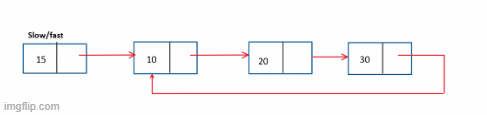After loop detection, we move our slow pointer to the head and then move both slow and the fast pointers at the same speed and check if the next pointer of the slow and fast pointer are the same.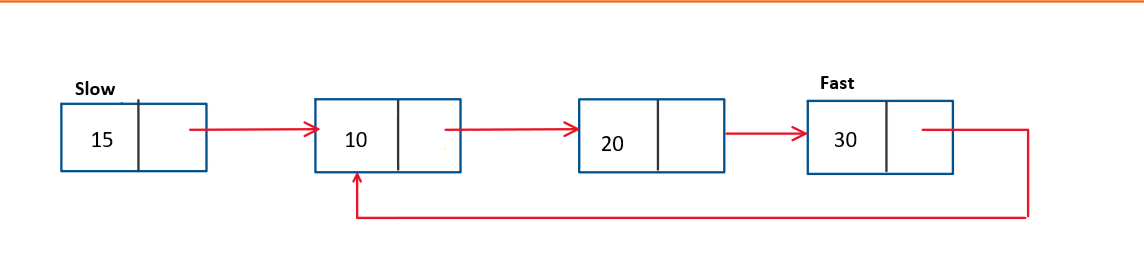In this case, the next pointer of both slow and fast pointers are the same, so we are changing the next pointer of fast to point to NULL.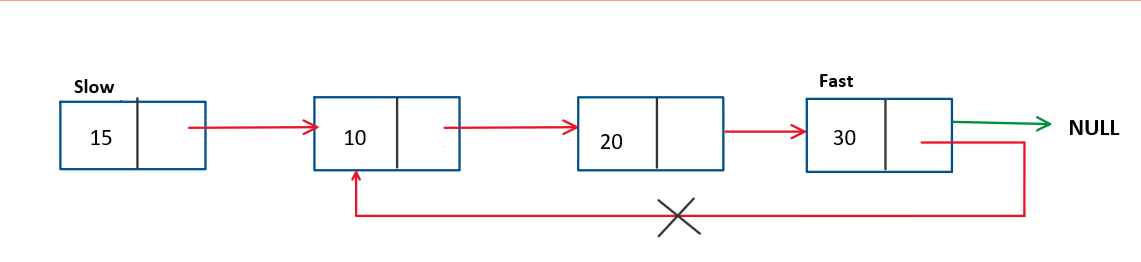For more understanding of how to detect loops in the linked list, refer to this article.

Special thanks to Yash Mishra for contributing to this article on takeUforward. If you also wish to share your knowledge with the takeUforward fam, please check out this article